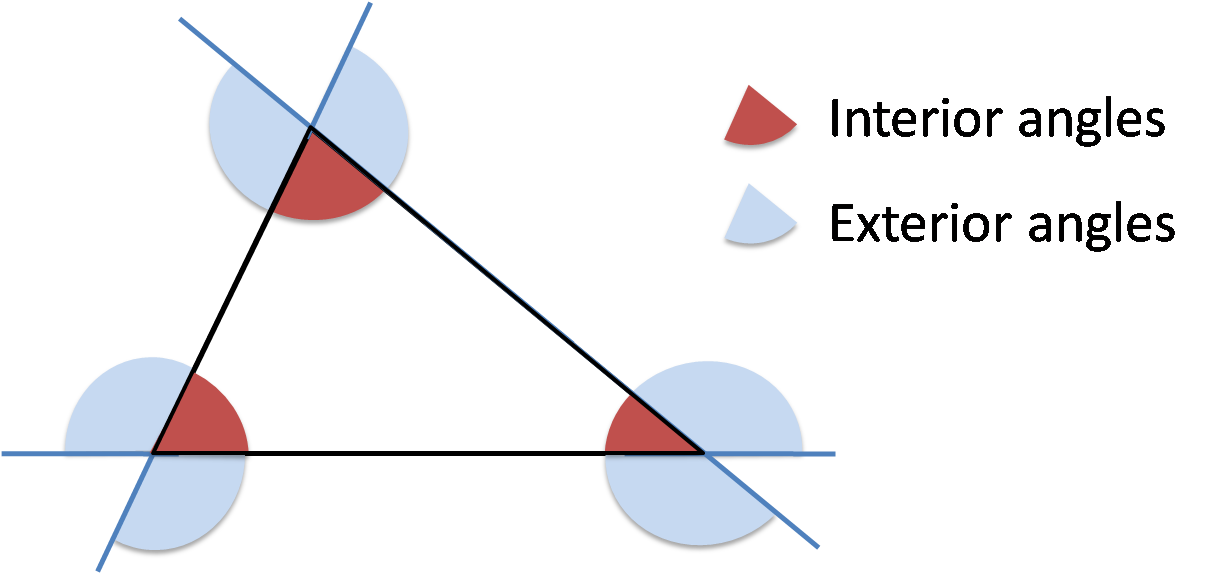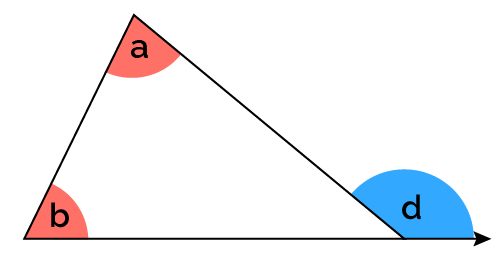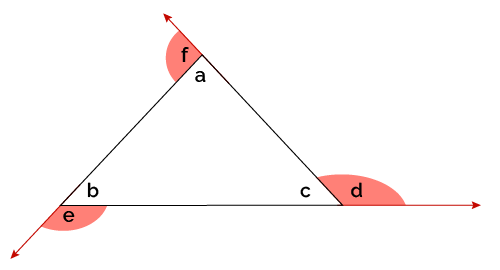# Exterior Angles of a Triangle Calculator

Created by Mariamy Chrdileli
Reviewed by Julia Żuławińska
Last updated: Jun 20, 2022

Welcome to the exterior angles of a triangle calculator, a simple and convenient tool that will help you calculate a triangle's exterior angles. If you are wondering how to find an exterior angle of a triangle, you're at the right place! Come along, and learn about the exterior angle theorem.

## How do I calculate exterior angles of a triangle?Let's start from the beginning - what is an exterior angle? If you extend any side of a triangle, the angle that is "outside" of the triangle, adjacent to an interior angle, is called an exterior angle. Each triangle has six exterior angles, where two angles at each vertex are equal.

According to the exterior angle theorem, the exterior angle is equal to the sum of the two opposite interior angles of the triangle.For example, if you wanted to determine the exterior angle d, you would use the following formula:

d = a + b

Keep in mind that the sum of exterior angles (taken one at each vertex) always equals 360°.In other words, d + e + f = 360°.

## How does the exterior angle calculator work?

Using the exterior angles of a triangle calculator is relatively easy.

Just enter values of two interior angles opposite of an exterior angle of your interest. The exterior angle theorem calculator will not only determine the value of an exterior angle you're looking for, but also other exterior angles of your triangle!

If you liked the exterior angles of a triangle calculator, come along, we have other related calculators that you may find helpful:

## FAQ

### What is the sum of the 3 exterior angles of a triangle?

The sum of exterior angles of a triangle, taken one at each vertex, always equals 360°. On the other hand, the sum of interior angles of a triangle equals 180°.

### What is the value of an exterior angle in a triangle if opposite interior angles are 40° and 75°?

If you want to calculate the value of an exterior angle if opposite interior angles are 40° and 75°, you can follow the following steps:

1. Add 40 to 74; in other words, sum up the interior angles of your triangle opposite the exterior angle of your interest.
2. That's all! You have determined the value of the exterior angle of your interest.
Mariamy Chrdileli
Interior anglesa
deg
b
deg
c
deg
Exterior angles
d
deg
e
deg
f
deg
People also viewed…

### Isosceles triangle angles

The isosceles triangle angles calculator can determine the lengths of an isosceles triangle's legs from its angles, and vice versa.

### Podcasts

Do you feel like you could be doing something more productive or educational while on a bus? Or while cleaning the house? Well, why don't you dive into the rich world of podcasts! With this podcast calculator, we'll work out just how many great interviews or fascinating stories you can go through by reclaiming your 'dead time'!

### SSA triangle

Use our SSA triangle calculator to solve the ambiguous case of the law of sines.

### Steps to calories

Steps to calories calculator helps you to estimate the total amount to calories burned while walking.